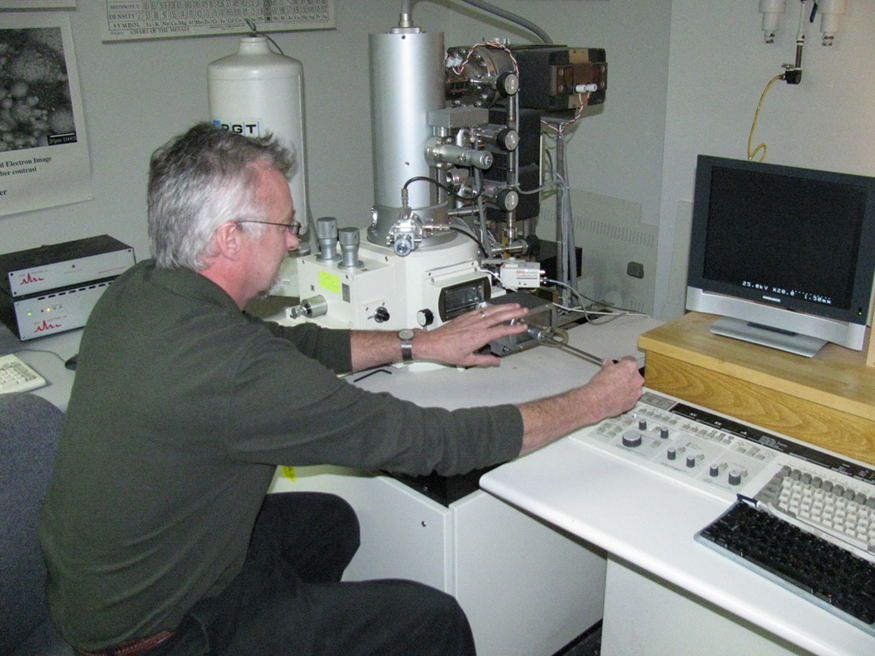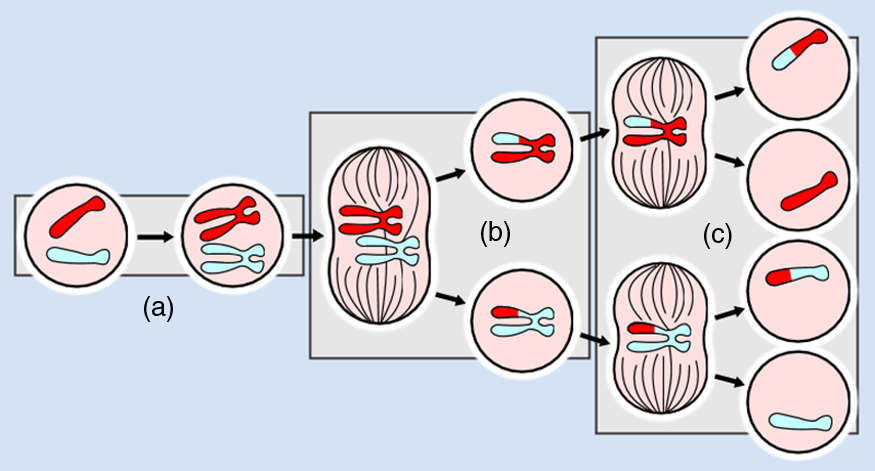# 26.4 Microscopes  (Page 5/9)

 Page 5 / 9An electron microscope has the capability to image individual atoms on a material. The microscope uses vacuum technology, sophisticated detectors and state of the art image processing software. (credit: Dave Pape)The image shows a sequence of events that takes place during meiosis. (credit: PatríciaR, Wikimedia Commons; National Center for Biotechnology Information)

## Take-home experiment: make a lens

Look through a clear glass or plastic bottle and describe what you see. Now fill the bottle with water and describe what you see. Use the water bottle as a lens to produce the image of a bright object and estimate the focal length of the water bottle lens. How is the focal length a function of the depth of water in the bottle?

## Test prep for ap courses

Which of the following correctly describes the image created by a microscope?

1. The image is real, inverted, and magnified.
2. The image is virtual, inverted, and magnified.
3. The image is real, upright, and magnified.
4. The image is virtual, upright, and magnified.

(b)

Use the diagram shown below to answer the following questions.

Draw two rays leaving the arrow shown to the left of both lenses. Use ray tracing to draw the images created by the objective and eyepiece lenses. Label the images as i o and i e .

## Section summary

• The microscope is a multiple-element system having more than a single lens or mirror.
• Many optical devices contain more than a single lens or mirror. These are analysed by considering each element sequentially. The image formed by the first is the object for the second, and so on. The same ray tracing and thin lens techniques apply to each lens element.
• The overall magnification of a multiple-element system is the product of the magnifications of its individual elements. For a two-element system with an objective and an eyepiece, this is
$m={m}_{\text{o}}{m}_{\text{e}}\text{,}$
where ${m}_{\text{o}}$ is the magnification of the objective and ${m}_{\text{e}}$ is the magnification of the eyepiece, such as for a microscope.
• Microscopes are instruments for allowing us to see detail we would not be able to see with the unaided eye and consist of a range of components.
• The eyepiece and objective contribute to the magnification. The numerical aperture $\left(\text{NA}\right)$ of an objective is given by
$\text{NA}=n\phantom{\rule{0.25em}{0ex}}\text{sin}\phantom{\rule{0.25em}{0ex}}\alpha$
where $n$ is the refractive index and $\alpha$ the angle of acceptance.
• Immersion techniques are often used to improve the light gathering ability of microscopes. The specimen is illuminated by transmitted, scattered or reflected light though a condenser.
• The $f/#$ describes the light gathering ability of a lens. It is given by
$f/#=\frac{f}{D}\approx \frac{1}{2\mathrm{NA}}.$

## Conceptual questions

Geometric optics describes the interaction of light with macroscopic objects. Why, then, is it correct to use geometric optics to analyse a microscope’s image?

The image produced by the microscope in [link] cannot be projected. Could extra lenses or mirrors project it? Explain.

Why not have the objective of a microscope form a case 2 image with a large magnification? (Hint: Consider the location of that image and the difficulty that would pose for using the eyepiece as a magnifier.)

What advantages do oil immersion objectives offer?

How does the $\text{NA}$ of a microscope compare with the $\text{NA}$ of an optical fiber?

## Problem exercises

A microscope with an overall magnification of 800 has an objective that magnifies by 200. (a) What is the magnification of the eyepiece? (b) If there are two other objectives that can be used, having magnifications of 100 and 400, what other total magnifications are possible?

(a) 4.00

(b) 1600

(a) What magnification is produced by a 0.150 cm focal length microscope objective that is 0.155 cm from the object being viewed? (b) What is the overall magnification if an $8×$ eyepiece (one that produces a magnification of 8.00) is used?

(a) Where does an object need to be placed relative to a microscope for its 0.500 cm focal length objective to produce a magnification of $–400$ ? (b) Where should the 5.00 cm focal length eyepiece be placed to produce a further fourfold (4.00) magnification?

(a) 0.501 cm

(b) Eyepiece should be 204 cm behind the objective lens.

You switch from a $1.40\text{NA}\phantom{\rule{0.25em}{0ex}}\text{60}×$ oil immersion objective to a $1.40\text{NA}\phantom{\rule{0.25em}{0ex}}\text{60}×$ oil immersion objective. What are the acceptance angles for each? Compare and comment on the values. Which would you use first to locate the target area on your specimen?

An amoeba is 0.305 cm away from the 0.300 cm focal length objective lens of a microscope. (a) Where is the image formed by the objective lens? (b) What is this image’s magnification? (c) An eyepiece with a 2.00 cm focal length is placed 20.0 cm from the objective. Where is the final image? (d) What magnification is produced by the eyepiece? (e) What is the overall magnification? (See [link] .)

(a) +18.3 cm (on the eyepiece side of the objective lens)

(b) -60.0

(c) -11.3 cm (on the objective side of the eyepiece)

(d) +6.67

(e) -400

You are using a standard microscope with a $0.10\text{NA}\phantom{\rule{0.25em}{0ex}}\text{4}×$ objective and switch to a $0.65\text{NA}\phantom{\rule{0.25em}{0ex}}\text{40}×$ objective. What are the acceptance angles for each? Compare and comment on the values. Which would you use first to locate the target area on of your specimen? (See [link] .)

Unreasonable Results

Your friends show you an image through a microscope. They tell you that the microscope has an objective with a 0.500 cm focal length and an eyepiece with a 5.00 cm focal length. The resulting overall magnification is 250,000. Are these viable values for a microscope?

what is angular velocity
Why does earth exert only a tiny downward pull?
hello
Islam
Why is light bright?
an 8.0 capacitor is connected by to the terminals of 60Hz whoes rms voltage is 150v. a.find the capacity reactance and rms to the circuit
thanks so much. i undersooth well
what is physics
is the study of matter in relation to energy
Kintu
a submersible pump is dropped a borehole and hits the level of water at the bottom of the borehole 5 seconds later.determine the level of water in the borehole
what is power?
power P = Work done per second W/ t. It means the more power, the stronger machine
Sphere
e.g. heart Uses 2 W per beat.
Rohit
A spherica, concave shaving mirror has a radius of curvature of 32 cm .what is the magnification of a persons face. when it is 12cm to the left of the vertex of the mirror
did you solve?
Shii
1.75cm
Ridwan
my name is Abu m.konnek I am a student of a electrical engineer and I want you to help me
Abu
the magnification k = f/(f-d) with focus f = R/2 =16 cm; d =12 cm k = 16/4 =4
Sphere
what do we call velocity
Kings
A weather vane is some sort of directional arrow parallel to the ground that may rotate freely in a horizontal plane. A typical weather vane has a large cross-sectional area perpendicular to the direction the arrow is pointing, like a “One Way” street sign. The purpose of the weather vane is to indicate the direction of the wind. As wind blows pa
hi
Godfred
Godfred
If a prism is fully imersed in water then the ray of light will normally dispersed or their is any difference?
the same behavior thru the prism out or in water bud abbot
Ju
If this will experimented with a hollow(vaccum) prism in water then what will be result ?
Anurag
What was the previous far point of a patient who had laser correction that reduced the power of her eye by 7.00 D, producing a normal distant vision power of 50.0 D for her?
What is the far point of a person whose eyes have a relaxed power of 50.5 D?
Jaydie
What is the far point of a person whose eyes have a relaxed power of 50.5 D?
Jaydie
A young woman with normal distant vision has a 10.0% ability to accommodate (that is, increase) the power of her eyes. What is the closest object she can see clearly?
Jaydie
29/20 ? maybes
Ju
In what ways does physics affect the society both positively or negatively
how can I read physics...am finding it difficult to understand...pls help
try to read several books on phy don't just rely one. some authors explain better than other.
Ju
And don't forget to check out YouTube videos on the subject. Videos offer a different visual way to learn easier.
Ju
hope that helps
JuByByBy OpenStaxBy OpenStaxBy RhodesBy RhodesBy Sebastian Sieczko...By Robert MurphyBy Brooke DelaneyBy Stephen VoronBy David CoreyBy Donyea Sweets